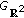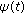# Existence and Stability of Steady Waves for the Hasegawa-Mima Equation

## Abstract

By introducing a compactness lemma and considering a constrained variational problem, we obtain a setof steady waves for Hasegawa-Mima equation, which describes the motion of drift waves in plasma. Moreover, we prove thatis a stable set for the initial value problem of the equation, in the sense that a solutionwhich starts nearwill remain near it for all time.

## Author information

Authors

### Corresponding author

Correspondence to Daiwen Huang.

## Rights and permissions

Reprints and Permissions

Guo, B., Huang, D. Existence and Stability of Steady Waves for the Hasegawa-Mima Equation. Bound Value Probl 2009, 509801 (2009). https://doi.org/10.1155/2009/509801

• Accepted:

• Published:

• DOI: https://doi.org/10.1155/2009/509801

### Keywords

• Differential Equation
• Partial Differential Equation
• Ordinary Differential Equation
• Functional Equation